M362K Third Midterm Exam, given on April 9, 2001

(Solutions below.)

Problem 1. Monday Blues

A word is chosen at random from the (undoubtedly true) sentence ``I hate to take math tests on Mondays''. That is, each word has an equal chance of being chosen. Let X be the number of letters in the word. Let Y be the number of vowels in the word (yes, the ``y'' in Mondays counts as a vowel).

a) Write down the pdf of X (that is,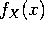).

b) Compute the expectation E(X).

c) Write down the joint pdf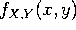.

d) Are the events X=4 and Y=2 independent? Are the events X=6 and Y=2 independent? Are X and Y independent random variables?

Problem 2. Joint distributions

Let X and Y be independent continuous random variables, each chosen uniformly in the interval [0,1]. That is,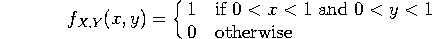Let Z be the larger of X and Y. That is,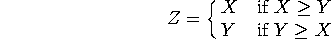a) What is the probability that (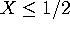and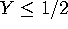)?

b) Compute the cumulative distribution function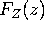.

c) Compute the probability density function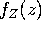.

d) Compute the expectation E(Z).

A random variable X has cumulative distribution function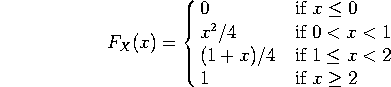(You may find it helpful to sketch this function).

a) What is the probability that X=0? What is the probability that X=1? What is the probability that X=2?

b) What is the probability that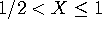?

c) What is the probability that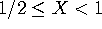?

d) What is the probability that X > 1.5

Problem 4. Manipulating random variables

Let X be continuously distributed between 1 and e with pdf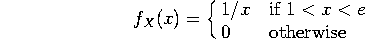a) Compute E(X).

b) Compute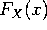.

c) Let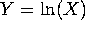. Compute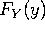, and from it compute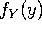.

d) Compute E(Y). [There are two ways to do this. One uses the results of part (b). The other does not.]

SOLUTIONS

Problem 1. Monday Blues

A word is chosen at random from the (undoubtedly true) sentence ``I hate to take math tests on Mondays''. That is, each word has an equal chance of being chosen. Let X be the number of letters in the word. Let Y be the number of vowels in the word (yes, the ``y'' in Mondays counts as a vowel).

a) Write down the pdf of X (that is,).

There are 8 words in all, one with one letter and one vowel (I) , two with two letters and one vowel (to, on), one with four letters and one vowel (math), two with four letters and two vowels (hate, take), one with 5 letters and one vowel (tests) and one with 7 letters and 3 vowels (Mondays). Since each word has an equal probability, the joint pdf is given in the following table: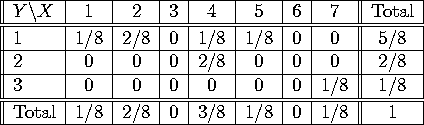The marginal pdf for X can be read off the bottom.

b) Compute the expectation E(X).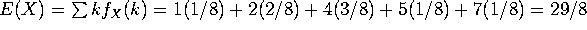.

c) Write down the joint pdf.

(see above)

d) Are the events X=4 and Y=2 independent? Are the events X=6 and Y=2 independent? Are X and Y independent random variables?

P(X=4 and Y=2)=2/8, while P(X=4)=3/8 and P(Y=2)=2/8. Since 2/8 is not equal to 3/8 times 2/8, the events X=4 and Y=2 are not independent.

P(X=6)=0, P(Y=2)=2/8, and P(X=6 and Y=2)=0, which does equal 0 times 2/8, so these events ARE independent.

Since X=4 and Y=2 are not independent events, X and Y are not independent random variables.

Problem 2. Joint distributions

Let X and Y be independent continuous random variables, each chosen uniformly in the interval [0,1]. That is,Let Z be the larger of X and Y. That is,a) What is the probability that (and)?

Since X and Y are independent, P(X < 1/2 and Y < 1/2)=P(X < 1/2) P(Y < 1/2) = P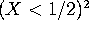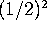= 1/4.  (I suppose one should say "less than or equal to 1/2", rather than "< 1/2", but for a continuous distribution it doesn't matter).

b) Compute the cumulative distribution function.

Since Z is the larger of X and Y,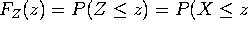and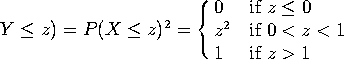c) Compute the probability density function.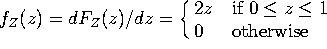d) Compute the expectation E(Z).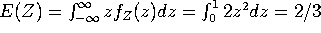.

A random variable X has cumulative distribution function(You may find it helpful to sketch this function).

a) What is the probability that X=0? What is the probability that X=1? What is the probability that X=2?

P(X=0) = (jump inat x=0) = 0.
P(X=1) = (jump inat x=1) = 1/4.
P(X=2) = (jump inat x=2) = 1/4.

b) What is the probability that?

P() =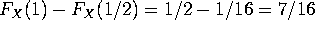.

c) What is the probability that?

This is the same as (b), plus the probability that X=1/2 (which is zero), minus the probability that X=1 (which is 1/4), that is 7/16 + 0 - 1/4 = 3/16.

d) What is the probability that X >1.5

This is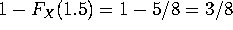.

Problem 4. Manipulating random variables

Let X be continuously distributed between 1 and e with pdfa) Compute E(X).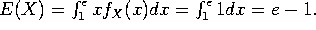b) Compute.

This is zero if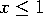and one if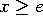. If 1 < x < e, then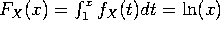.

c) Let. Compute, and from it compute.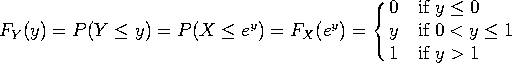.
In other words, Y is uniformly distributed between 0 and 1.

d) Compute E(Y). [There are two ways to do this. One uses the results of part (b). The other does not.]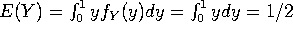, or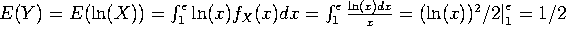.